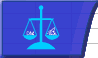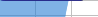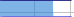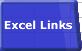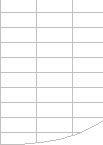# Controlling Excel CalculationSmart Recalculation Evaluation Circumstances Calculation Process Dependency Trees Controlling Calculation "CALCULATE" in Status Bar Calculation Methods Calculating from VBA Volatile Functions & Actions User-Defined Functions Repetitive Calculation Version Timing Comparison### Controlling Calculation

Excel has a range of options allowing you to control the way it calculates. You can change these options using the Tools -->Options-->Calculation tab.

#### Calculation Settings Keep Changing

Because a number of Excel's calculation settings work at the application level (they are the same for all open workbooks), and are set by the first workbook opened, they may appear to change randomly depending on the sequence in which workbooks are opened. FastExcel Version 2 allows you to solve many of these problems.

#### Automatic Calculation.

Automatic calculation mode means that Excel will automatically recalculate all open workbooks at each and every change, and whenever you open a workbook.
Usually when you open a workbook in Automatic mode and Excel recalculates you will not see the recalculation because nothing will have changed since the workbook was saved.
An exception is when you open a workbook in Excel 2000 that was saved using Excel 97, or you open using Excel2002/2003 a workbook saved in Excel2000: because the Excel calculation engines are different a Full calculation is done.

#### Manual Calculation.

 Press F9 if the status bar shows Calculate Manual calculation mode means that Excel will only recalculate all open workbooks when you request it by pressing F9 or Ctrl-Alt-F9, or when you Save a workbook. For workbooks taking more than a fraction of a second to recalculate it is usually better to set Calculation to Manual. Excel tells you when the workbook needs recalculation by showing Calculate in the status bar. If this message wont disappear see Status bar shows Calculate .

#### Automatic except Tables.

Excels Data Tables feature is designed to do multiple calculations of the workbook, each driven by different values in the table. So using Automatic except Tables will stop Excel from automatically triggering the multiple calculations at each calculation, but will still calculate all dependent formulae except tables.

#### Application level settings.

The following calculation settings are held at the Application level rather than for each workbook, so they apply to all open workbooks.

• Calculation Mode (Automatic, Automatic except Tables, Manual, Calculate before Save).
• Iteration settings (Iteration On/Off, Max Iterations, Max Change).

Rather confusingly these application level settings are saved in each workbook, but only the settings in the first workbook opened are actioned: the settings in subsequent workbooks are ignored.

#### Workbook level settings.

The following calculation settings are held at workbook level, so each open workbook can have different settings.

• Update remote references.
• Precision as displayed.
• 1904 date system.
• Accept labels in formulae.

All these workbook level calculation settings are saved and restored with the workbook, except Update remote references.

#### Controlling Excels Calculation Options.

FastExcel Version 2 significantly extends Excel’s calculation options. See the FastExcel Version 2 User Guide for details.
The details below refer to Excel’s standard calculation options.

 Calculation Mode operates at Application rather than Workbook level. When Excel has no workbooks open, or when you start Excel, it sets the initial calculation mode and settings from the first non-template, non-addin, non-Personal.xls workbook that you open This means that the calculation mode setting in subsequently opened workbooks will be ignored, although you can change the mode yourself at any time using Tools-->Options-->Calculation. As soon as you change the calculation mode, it applies to all subsequently opened workbooks. If you want to override the way Excel sets the initial calculation mode you can set it yourself by creating a module in ThisWorkbook (doubleclick ThisWorkbook in the Project Explorer window in the VBE), and adding this code. This example sets calculation to Manual. Note that this will NOT prevent the workbook being recalculated if it is opened in Automatic mode. Private Sub Workbook_Open()    Application.Calculation = xlCalculationManual End Sub If calculation is set to Automatic when a workbook containing this code is opened, Excel will start the recalculation process before the Open event is executed. You can bypass this problem by: Using FastExcel version 2's Initial Calculation setting. Have a single workbook (as long as its not called Personal.xls) in your XLSTART directory which has been saved in Manual mode. Have an Addin installed which sets calculation to manual in its WorkBook_Open or Auto_Open procedures (Excel 97 and Excel 2000 only). If you have a workbook that was saved in Automatic mode, but you want to open it in Manual mode: Open Excel with a blank workbook. Switch to Manual (Tools-->Options-->Calculation). Open the workbook that was saved in Automatic. If you have a workbook that was saved in Manual mode, but you want to open it in Automatic Mode: Open Excel with a blank workbook. Switch to Manual (Tools-->Options-->Calculation). Then switch back to Automatic. The two steps are required to lock in the mode change. Open the workbook that was saved in Manual.

#### Excluding Worksheets from Recalculation.

You can prevent worksheets from being calculated or recalculated by using VBA to set the Worksheet.EnableCalculation property to False. See Calculating from VBA.
Note that this setting is NOT saved with the workbook.

FastExcel Version 2 gives you much better control over which worksheets are calculated using FastExcel MixMode settings.

#### Recalculate before Save

In Manual mode this checkbox controls whether Excel will recalculate the workbook as part of the save process. The default is Yes.

#### Iteration

 Usually it pays to un-check the Iteration box. If you have intentional circular references in your workbook, these settings allow you to control the maximum number of times the workbook will be recalculated (iterations) and the convergence criteria (maximum change: when to stop). The default should be to un-check the iteration box so that Excel does not try to solve accidental circular references.

#### Update Remote References.

If TRUE Automatically update any remote references (DDE Links to other programs) whenever Excel recalculates.

#### Precision as Displayed.

Checking this box will force Excel to calculate to the number of decimal places that appear as a result of your formatting, and will permanently change any numbers stored in cells.
You need to be sure you have thought through the full implications of this before using it.
Precision as Displayed slows down calculation.

#### 1904 Date System.

If TRUE changes the starting date from which all dates are calculated from January 1 1900 to January 2 1904.© 2001-2020 Decision Models Legal Disclaimer Privacy Statement